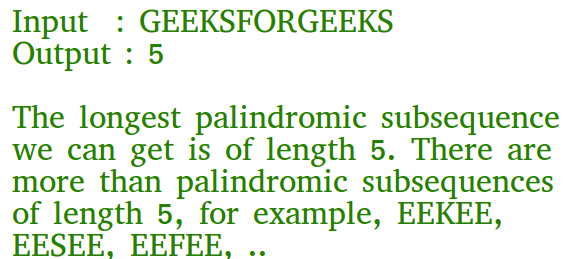# C# Program for Longest Palindromic Subsequence | DP-12

• Last Updated : 13 Aug, 2021

Given a sequence, find the length of the longest palindromic subsequence in it.As another example, if the given sequence is “BBABCBCAB”, then the output should be 7 as “BABCBAB” is the longest palindromic subsequence in it. “BBBBB” and “BBCBB” are also palindromic subsequences of the given sequence, but not the longest ones.

1) Optimal Substructure:
Let X[0..n-1] be the input sequence of length n and L(0, n-1) be the length of the longest palindromic subsequence of X[0..n-1].
If last and first characters of X are same, then L(0, n-1) = L(1, n-2) + 2.
Else L(0, n-1) = MAX (L(1, n-1), L(0, n-2)).

Following is a general recursive solution with all cases handled.

## C#

 `// C# program of above approach``using` `System;` `public` `class` `GFG {` `    ``// A utility function to get max of two integers``    ``static` `int` `max(``int` `x, ``int` `y)``    ``{``        ``return` `(x > y) ? x : y;``    ``}``    ``// Returns the length of the longest palindromic subsequence in seq` `    ``static` `int` `lps(``char``[] seq, ``int` `i, ``int` `j)``    ``{``        ``// Base Case 1: If there is only 1 character``        ``if` `(i == j) {``            ``return` `1;``        ``}` `        ``// Base Case 2: If there are only 2 characters and both are same``        ``if` `(seq[i] == seq[j] && i + 1 == j) {``            ``return` `2;``        ``}` `        ``// If the first and last characters match``        ``if` `(seq[i] == seq[j]) {``            ``return` `lps(seq, i + 1, j - 1) + 2;``        ``}` `        ``// If the first and last characters do not match``        ``return` `max(lps(seq, i, j - 1), lps(seq, i + 1, j));``    ``}` `    ``/* Driver program to test above function */``    ``public` `static` `void` `Main()``    ``{``        ``String seq = ``"GEEKSFORGEEKS"``;``        ``int` `n = seq.Length;``        ``Console.Write(``"The length of the LPS is "` `+ lps(seq.ToCharArray(), 0, n - 1));``    ``}``}` `// This code is contributed by Rajput-Ji`

Output:

`The length of the LPS is 5`

Dynamic Programming Solution

## C#

 `// A Dynamic Programming based C# Program``// for the Egg Dropping Puzzle``using` `System;` `class` `GFG {` `    ``// A utility function to get max of``    ``// two integers``    ``static` `int` `max(``int` `x, ``int` `y)``    ``{``        ``return` `(x > y) ? x : y;``    ``}` `    ``// Returns the length of the longest``    ``// palindromic subsequence in seq``    ``static` `int` `lps(``string` `seq)``    ``{``        ``int` `n = seq.Length;``        ``int` `i, j, cl;` `        ``// Create a table to store results``        ``// of subproblems``        ``int``[, ] L = ``new` `int``[n, n];` `        ``// Strings of length 1 are``        ``// palindrome of length 1``        ``for` `(i = 0; i < n; i++)``            ``L[i, i] = 1;` `        ``// Build the table. Note that the``        ``// lower diagonal values of table``        ``// are useless and not filled in``        ``// the process. The values are``        ``// filled in a manner similar to``        ``// Matrix Chain Multiplication DP``        ``// solution (See``        ``// https:// www.geeksforgeeks.org/matrix-chain-multiplication-dp-8/``        ``// cl is length of substring``        ``for` `(cl = 2; cl <= n; cl++) {``            ``for` `(i = 0; i < n - cl + 1; i++) {``                ``j = i + cl - 1;` `                ``if` `(seq[i] == seq[j] && cl == 2)``                    ``L[i, j] = 2;``                ``else` `if` `(seq[i] == seq[j])``                    ``L[i, j] = L[i + 1, j - 1] + 2;``                ``else``                    ``L[i, j] = max(L[i, j - 1], L[i + 1, j]);``            ``}``        ``}` `        ``return` `L[0, n - 1];``    ``}` `    ``/* Driver program to test above``    ``functions */``    ``public` `static` `void` `Main()``    ``{``        ``string` `seq = ``"GEEKS FOR GEEKS"``;``        ``int` `n = seq.Length;``        ``Console.Write(``"The length of the "``                      ``+ ``"lps is "` `+ lps(seq));``    ``}``}` `// This code is contributed by nitin mittal.`

Output

`The length of the lps is 7`

Please refer complete article on Longest Palindromic Subsequence | DP-12 for more details!

My Personal Notes arrow_drop_up